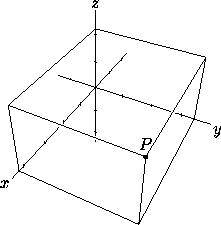A# Cartesian coordinatesCartesian coordinates are an ordered set of real numbers that defines the position of a point in terms of its projection on to mutually perpendicular number lines. In the plane, each point is defined by two such projections, one on to the x-axis (the abscissa) and one on to the y-axis (the ordinate), and is written as an ordered pair of real numbers (x, y). The same system works equally in spaces of three or more dimensions.

Cartesian coordinates are the most common system of rectangular coordinates employed in analytical geometry. The term is named after René Descartes.